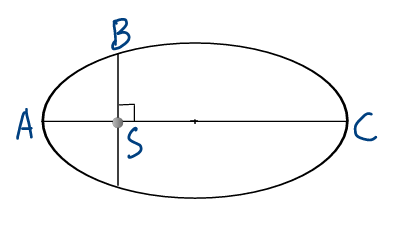# How To Solve NEET 2018 With A Smile – II

### Let Us YOU Solve NEET 2018

In this blog, we continue to discuss more Physics MCQs from NEET 2018 Paper. Subscribe to PhysicsAcademyOnline.com., and gain access to a large number of video lectures depending on your specific need. Apart from the hint, every question we discuss is accompanied by a video solution to confirm your final answer.

Question 152:  A solid sphere is in rolling motion. In rolling motion, a body possesses transnational kinetic energy, Kt , as well as rotational kinetic energy, Kr , simultaneously. The ratio Kt : (Kt + Kr) for the sphere is:

(1) 7 : 10                (2) 2 : 5                 (3) 10: 7                 (4) 5:7

What is the transnational kinetic energy, Kt , of a rolling body? What is the rotational kinetic energy, Kr , of a rolling body? Watch the lecture: Chapter Name – Mechanics; Category –  Basic; Topic Name – Rotational Mechanics; Video Name – Rotational Kinetic Energy, and Comparison between Translation and Rotation.

What is the moment of inertia of a solid sphere about (an axis through) centre of mass? Pick the formula from here:   Chapter Name – Mechanics; Category –  Basic; Topic Name – Rotational Mechanics; Video Name – Calculation of Moment of Inertia for Various Bodies II.

What is the nonslip condition for velocity of a rolling body? Follow this: Chapter Name – Mechanics; Category –  Basic; Topic Name – Rotational Mechanics; Video Name – Rolling Motion of a Rigid Body on Horizontal Floor.

Express Kr in a form comparable to Kt . The required ratio comes out.

Question 153:  The kinetic energies of a planet in an elliptical orbit about the Sun at positions A, B and C are KA , KB and KC , respectively. AC is the major axis and SB is perpendicular to AC at the position of the Sun, S. See figure. Then:(1) KA < KB < KC
(2) KB > KA > KC
(3) KB < KA < KC
(4) KA > KB > KC

What is Kepler’s second law? What does it imply about linear speed of a planet at perihelion (point A, closest to the sun) and at aphelion (point C, farthest from the sun)? Lucidly explained here: Chapter Name – Mechanics; Category –  Basic; Topic Name – Gravitation; Video Name – Kepler’s Laws of Planetary Motion.

You need nothing more.

Question 154:  If the mass of the Sun were 10 times smaller and the universal gravitational constant were 10 times larger in magnitude, which of the following is not correct:

(1) Raindrops will fall faster

(2) “g” on the Earth will not change

(3) Time period of a simple pendulum on the Earth will decrease

(4) Walking on the ground will become more difficult

We have already seen the benefit of a shortcut in MCQs. You can try that here as well. Out of the four options, which one looks “different”? How?

What is the formula for acceleration due to gravity, g, on the Earth’s surface? Study this: Chapter Name – Mechanics; Category –  Basic; Topic Name – Gravitation; Video Name – Gravity.

Does the change in Sun’s mass affect g? Does the change in gravitational constant affect g?

Question 155:  A solid sphere is rotating freely about its axis of symmetry in free space. The radius of the sphere is increased keeping its mass same. Which of the following physical quantities would remain constant for the sphere?

(1) angular velocity   (2) angular momentum   (3) rotational kinetic energy   (4) moment of inertia

Another opportunity to take a shortcut! How do you interpret “free rotation in free space”? Which physical quantity is absent and, in turn, which quantity remains constant? Learn an important principle from: Chapter Name – Mechanics; Category –  Basic; Topic Name – Rotational Mechanics; Video Name – Principle of Conservation of Angular Momentum, and Its Applications.

You already found the correct option. Just for the sake of completeness, can you argue why the other three physical quantities change?